Next: Rules of probability Up: Bayesian Inference in Processing Previous: Introduction

# Uncertainty and probability

In the practice of science, we constantly find ourselves in a state of uncertainty. Uncertainty about the data that an experiment shall yield. Uncertainty about the true value of a physical quantity, even after an experiment has been done. Uncertainty about model parameters, calibration constants, and other quantities that might influence the outcome of the experiment, and hence influence our conclusions about the quantities of interest, or the models that might have produced the observed results.

In general, we know through experience that not all the events that could happen, or all conceivable hypotheses, are equally likely. Let us consider the outcome of you measuring the temperature at the location where you are presently reading this paper, assuming you use a digital thermometer with one degree resolution (or you round the reading at the degree if you have a more precise instrument). There are some values of the thermometer display you are more confident to read, others you expect less, and extremes you do not believe at all (some of them are simply excluded by the thermometer you are going to use). Given two events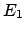and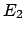, for example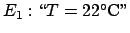and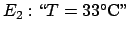, you might considermuch more probable than, just meaning that you believeto happen more than. We could use different expressions to mean exactly the same thing: you considermore likely; you are more confident in; having to choose betweenandto win a price, you would promptly choose; having to classify with a number, that we shall denote with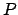, your degree of confidence on the two outcomes, you would write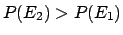; and many others.

On the other hand, we would rather state the opposite, i.e., with the same meaning of symbols and referring exactly to the same events: what you are going to read at your place with your thermometer. The reason is simply because we do not share the same status of information. We do not know who you are and where you are in this very moment. You and we are uncertain about the same event, but in a different way. Values that might appear very probable to you now, appear quite improbable, though not impossible, to us.

In this example we have introduced two crucial aspects of the Bayesian approach:

1. As it is used in everyday language, the term probability has the intuitive meaning of the degree of belief that an event will occur.''
2. Probability depends on our state of knowledge, which is usually different for different people. In other words, probability is unavoidably subjective.

At this point, you might find all of this quite natural, and wonder why these intuitive concepts go by the esoteric name Bayesian.' We agree! The fact is that the main thrust of statistics theory and practice during the 20century has been based on a different concept of probability, in which it is defined as the limit of the long-term relative frequency of the outcome of these events. It revolves around the theoretical notion of infinite ensembles of identical experiments.' Without entering an unavoidably long critical discussion of the frequentist approach, we simply want to point out that in such a framework, there is no way to introduce the probability of hypotheses. All practical methods to overcome this deficiency yield misleading, and even absurd, conclusions. See (D'Agostini 1999c) for several examples and also for a justification of why frequentistic test often work'.

Instead, if we recover the intuitive concept of probability, we are able to talk in a natural way about the probability of any kind of event, or, extending the concept, of any proposition. In particular, the probability evaluation based on the relative frequency of similar events occurred in the past is easily recovered in the Bayesian theory, under precise condition of validity (see Sect. 5.3). Moreover, a simple theorem from probability theory, Bayes' theorem, which we shall see in the next section, allows us to update probabilities on the basis of new information. This inferential use of Bayes' theorem is only possible if probability is understood in terms of degree of belief. Therefore, the terms Bayesian' and based on subjective probability' are practically synonyms,and usually mean in contrast to the frequentist, or conventional, statistics.' The terms Bayesian' and subjective' should be considered transitional. In fact, there is already the tendency among many Bayesians to simply refer to probabilistic methods,' and so on (Jeffreys 1961, de Finetti 1974, Jaynes 1998 and Cowell et al 1999).

As mentioned above, Bayes' theorem plays a fundamental role in the probability theory. This means that subjective probabilities of logically connected events are related to each other by mathematical rules. This important result can be summed up by saying, in practical terms, that degrees of belief follow the same grammar as abstract axiomatic probabilities.' Hence, all formal properties and theorems from probability theory follow.

Within the Bayesian school, there is no single way to derive the basic rules of probability (note that they are not simply taken as axioms in this approach). de Finetti's principle of coherence (de Finetti 1974) is considered the best guidance by many leading Bayesians (Bernardo and Smith 1994, O'Hagan 1994, Lad 1996 and Coletti and Scozzafava 2002). See (D'Agostini 1999c) for an informal introduction to the concept of coherence, which in simple words can be outlined as follows. A person who evaluates probability values should be ready to accepts bets in either direction, with odd ratios calculated from those values of probability. For example, an analyst that declares to be confident 50% on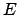should be aware that somebody could ask him to make a 1:1 bet onor on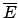. If he/she feels uneasy, it means that he/she does not consider the two events equally likely and the 50% was incoherent.'

Others, in particular practitioners close to the Jaynes' Maximum Entropy school (Jaynes 1957a, 1957b) feel more at ease with Cox's logical consistency reasoning, requiring some consistency properties (desiderata') between values of probability related to logically connected propositions. (Cox 1946). See also (Jaynes 1998, Sivia 1997, and Fröhner 2000, and especially Tribus 1969), for accurate derivations and a clear account of the meaning and role of information entropy in data analysis. An approach similar to Cox's is followed by Jeffreys (1961), another leading figure who has contributed a new vitality to the methods based on this new' point of view on probability. Note that Cox and Jeffreys were physicists. Remarkably, Schrödinger (1947a, 1947b) also arrived at similar conclusions, though his definition of event is closer to the de Finetti's one. [Some short quotations from (Schrödinger 1947a) are in order. Definition of probability: ...a quantitative measure of the strength of our conjecture or anticipation, founded on the said knowledge, that the event comes true''. Subjective nature of probability: Since the knowledge may be different with different persons or with the same person at different times, they may anticipate the same event with more or less confidence, and thus different numerical probabilities may be attached to the same event.'' Conditional probability: Thus whenever we speak loosely of the probability of an event,' it is always to be understood: probability with regard to a certain given state of knowledge.'']Next: Rules of probability Up: Bayesian Inference in Processing Previous: Introduction
Giulio D'Agostini 2003-05-13